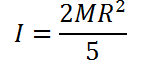# Moment of Inertia of a Solid Sphere Calculator

This CalcTown calculator calculates the moment of Inertia of a Solid Sphere along an axis passing through its centre.

kg
m

#### Result

Kg-m2where

M is the mass of the Sphere

R is the radius of the sphere.